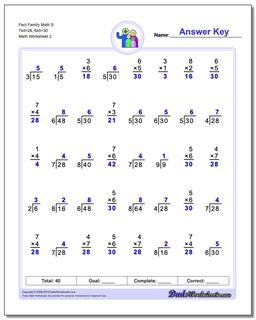One Dad. Four daughters. 9,251 worksheets... and counting!Fact Family Math S 7x4=28, 6x5=30 (Second Worksheet)

PropertyValue
DescriptionFact Family Math S 7x4=28, 6x5=30: These 40 problem multiplication and division fact family worksheets are perfect for either quick practice or a one minute timed test. For help on individual operations, check out the multiplication worksheets and division worksheets pages! (Second Worksheet)
Resource TypeWorksheet